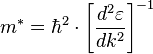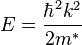# Effective mass question

## Homework Statement

Calculate the effective mass for a free electron.

## Homework Equations## The Attempt at a Solution

I know the above equation comes from, in the case of the free electron dispersion relation,.

However i'm not at all sure how to go about calculating it for a free electron, given that the two formulae are basically one in the same...

Note the following minor correction: the free electron dispersion relation is $\epsilon = \hbar ^2 k^2 /2m_e$. What happens when you plug this into the expression for the effective mass?